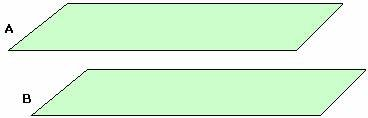Definition Of Parallel Planes

Planes that never intersect each other are known as Parallel Planes.

Planes which are not parallel are called intersecting planes and they always intersect in a line

Examples of Parallel PlanesThe above figure shows two parallel planes, A and B.
The opposite walls of a room, floor and ceiling are the examples of parallel planes.

Solved Example on Parallel Planes

Ques: Identify the parallel planes.A. RST and UZY
B. SXY and RUT
C. WZU and XYT
D. STU and XYT